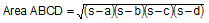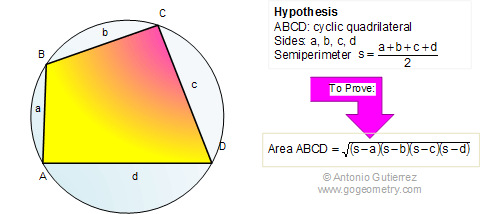Brahmagupta's Formula:  Area of cyclic quadrilateral. Level: High School, SAT Prep, College geometry If a cyclic quadrilateral ABCD has sides of lengths a, b, c, and d, and the semi-perimeter, then the area is given by:Brahmagupta (598–668) was an Indian mathematician and astronomer. Heron's formula is a special case of this formula, when one of the sides equal zero. The relationship between the general Brahmagupta's formula and the Heron's formula is similar to how the law of cosines extends the Pythagorean theorem.   See also: Brahmagupta's Theorem, Corollary,. Area Formula Extension.Home | Geometry | Areas | Brahmagupta | Classical Theorems | Email | By Antonio Gutierrez